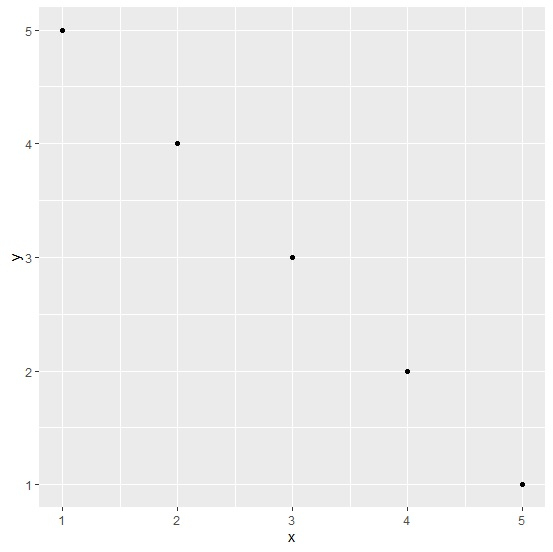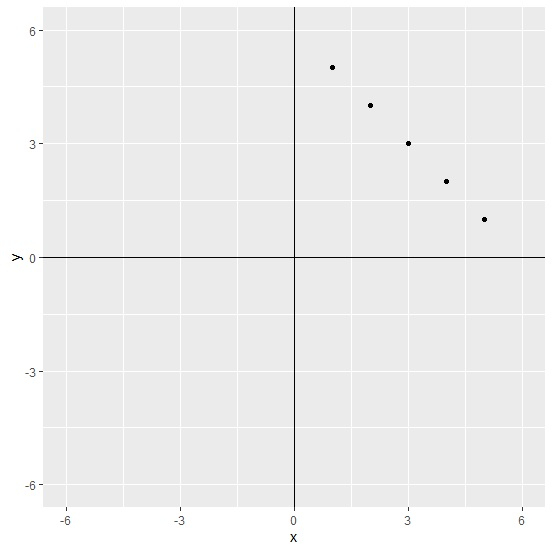# How to create a graph in R using ggplot2 with all the four quadrants?

The default graph created by using ggplot2 package shows the axes labels depending on the starting and ending values of the column of the data frame or vector but we might want to visualize it just like we do in paper form of graphs that shows all of the four quadrants. This can be done by using xlim, ylim, geom_hline, and geom_vline functions with ggplot function of ggplot2 package.

Consider the below data frame −

## Example

Live Demo

x<-1:5
y<-5:1
df<-data.frame(x,y)
df

## Output

  x y
1 1 5
2 2 4
3 3 3
4 4 2
5 5 1

## Example

library(ggplot2) ggplot(df,aes(x,y))+geom_point()

## OutputCreating point chart between x and y by showing all four quadrants −

## Example

ggplot(df,aes(x,y))+geom_point()+xlim(-6,6)+ylim(-6,6)+geom_hline(yintercept=0)+geom_vline(xintercept=0)

## Output